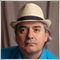# OrderClose not working414

Hi,

I have the following coding for my EA, please help me why the OrderClose is not triggerred? Do I need to use OrderSelect()? What is the purpose of OrderSelect()? Thank you.

```//+------------------------------------------------------------------+
//|                                                       chuale.mq4 |
//|                        Copyright 2014, MetaQuotes Software Corp. |
//|                                              http://www.abc.com |
//+------------------------------------------------------------------+
#property version   "1.00"
#property strict

extern double   TakeProfit=500;
extern double   Lots=0.1;
extern double   StopLoss=280;

double     bar1;
double     bar2;
double     bar3;
//+------------------------------------------------------------------+
//| Expert initialization function                                   |
//+------------------------------------------------------------------+
int init()
{

//---

//---
return(0);
}
//+------------------------------------------------------------------+
//| Expert deinitialization function                                 |
//+------------------------------------------------------------------+
int deinit()
{
//---

return(0);
}
//+------------------------------------------------------------------+
//| Expert tick function                                             |
//+------------------------------------------------------------------+
int start()
{
int ticket;
int total=OrdersTotal();
int    counted_bars=IndicatorCounted();
if(counted_bars>0) counted_bars--;
{
bar1=(iClose(Symbol(),PERIOD_M1,2)-iClose(Symbol(),PERIOD_M1,3))*100;
bar2=(iClose(Symbol(),PERIOD_M1,1)-iClose(Symbol(),PERIOD_M1,2))*100;
bar3=(iClose(Symbol(),PERIOD_M1,0)-iClose(Symbol(),PERIOD_M1,1))*100;
if (bar1+bar2>1)
{
if(total<1)
if (bar3<-1) OrderClose(OrderTicket(),OrderLots(),Bid,3,Green);
}
if (bar1+bar2<-1)
{
if(total<1)
ticket=OrderSend(Symbol(),OP_SELL,Lots,Bid,3,Bid+StopLoss*Point,Bid+-TakeProfit*Point,"Chua EA",12345,0,Red);
}
}

return(0);
//---
return(0);
}
//+------------------------------------------------------------------+
```3201

you dont have to use OrderSelect 4 OrderClose but u have 2 for OrderTicket(), OrderLots()414

qjol:
you dont have to use OrderSelect 4 OrderClose but u have 2 for OrderTicket(), OrderLots()

Dear qjol,

I dont quite understand what you mean, Could you please explain. Thank you3201

in ur code

`if (bar3>1)  OrderClose(OrderTicket(), OrderLots(),Ask,3,Red);`

u using OrderTicket() & OrderLots() & 4 these functions u must use OrderSelect()

As you can see in the documentation:

Note

The pool parameter is ignored if the order is selected by the ticket number. The ticket number is a unique order identifier.

To find out from what list the order has been selected, its close time must be analyzed. If the order close time equals to 0, the order is open or pending and taken from the terminal open positions list.

One can distinguish an open position from a pending order by the order type. If the order close time does not equal to 0, the order is a closed order or a deleted pending order and was selected from the terminal history. They also differ from each other by their order types.

The OrderSelect() function copies order data into program environment and all further calls of OrderClosePrice(), OrderCloseTime(), OrderComment(), OrderCommission(), OrderExpiration(), OrderLots(), OrderMagicNumber(), OrderOpenPrice(), OrderOpenTime(), OrderPrint(), OrderProfit(), OrderStopLoss(), OrderSwap(), OrderSymbol(), OrderTakeProfit(), OrderTicket(), OrderType() functions return the data, copied earlier. It means that in some cases the order details (open price, SL/TP levels or expiration date) may change and the data become non-actual. It is strongly recommended to call the OrderSelect() function before request the order data.414

So if i use the actual ticket number and Lots then no need to use OrderSelect, right? I only open one order at a time, may I knwo how to get the ticket number of the open order?

Thanks again6969

chuale:

So if i use the actual ticket number and Lots then no need to use OrderSelect, right? I only open one order at a time, may I knwo how to get the ticket number of the open order?

Thanks again

The OrderSend() funtion returns the Ticket number if succesful. I suggest you read the Book section on this site from begining to end to better understand how to use MQL, as well as consult the reference documentation for every function you use so as to better understand what parameters to use and what the return values are.414

Dear All,

```//+------------------------------------------------------------------+
//|                                                       chuale.mq4 |
//|                        Copyright 2014, MetaQuotes Software Corp. |
//|                                              http://www.abc.com |
//+------------------------------------------------------------------+
#property version   "1.00"
#property strict

extern double   TakeProfit=500;
extern double   Lots=0.1;
extern double   StopLoss=300;

double     bar1;
double     bar2;
double     bar3;
//+------------------------------------------------------------------+
//| Expert initialization function                                   |
//+------------------------------------------------------------------+
int init()
{

//---

//---
return(0);
}
//+------------------------------------------------------------------+
//| Expert deinitialization function                                 |
//+------------------------------------------------------------------+
int deinit()
{
//---

return(0);
}
//+------------------------------------------------------------------+
//| Expert tick function                                             |
//+------------------------------------------------------------------+
int start()
{
int ticket;
int total=OrdersTotal();
int    counted_bars=IndicatorCounted();
if(counted_bars>0) counted_bars--;
{
bar1=(iClose(Symbol(),PERIOD_M1,2)-iClose(Symbol(),PERIOD_M1,3))*100;
bar2=(iClose(Symbol(),PERIOD_M1,1)-iClose(Symbol(),PERIOD_M1,2))*100;
bar3=(iClose(Symbol(),PERIOD_M1,0)-iClose(Symbol(),PERIOD_M1,1))*100;
if (bar2>1)
{
if(total<1)
OrderSelect(1,SELECT_BY_POS);
if (bar3 <-1) OrderClose(OrderTicket(),Lots,Bid,3,Green);
}
if (bar2<-1)
{
if(total<1)
ticket=OrderSend(Symbol(),OP_SELL,Lots,Bid,3,Bid+StopLoss*Point,Bid+-TakeProfit*Point,"Chua EA",12345,0,Red);
}
}

return(0);
//---
return(0);
}
//+------------------------------------------------------------------+```3201

who told u that the no. of the order is 1 ?414

i only allow one open order at a time.414

Hi All,

Now I insert ticket and Lots in the OrderClose without using OrderTicket() and OrderLots() but it not closing the order too. Please help.

```//+------------------------------------------------------------------+
//|                                                       chuale.mq4 |
//|                        Copyright 2014, MetaQuotes Software Corp. |
//|                                              http://www.abc.com |
//+------------------------------------------------------------------+
#property version   "1.00"
#property strict

extern double   TakeProfit=500;
extern double   Lots=0.1;
extern double   StopLoss=300;

double     bar1;
double     bar2;
double     bar3;
//+------------------------------------------------------------------+
//| Expert initialization function                                   |
//+------------------------------------------------------------------+
int init()
{

//---

//---
return(0);
}
//+------------------------------------------------------------------+
//| Expert deinitialization function                                 |
//+------------------------------------------------------------------+
int deinit()
{
//---

return(0);
}
//+------------------------------------------------------------------+
//| Expert tick function                                             |
//+------------------------------------------------------------------+
int start()
{
int ticket;
int total=OrdersTotal();
int    counted_bars=IndicatorCounted();
if(counted_bars>0) counted_bars--;
{
bar1=(iClose(Symbol(),PERIOD_M1,2)-iClose(Symbol(),PERIOD_M1,3))*100;
bar2=(iClose(Symbol(),PERIOD_M1,1)-iClose(Symbol(),PERIOD_M1,2))*100;
bar3=(iClose(Symbol(),PERIOD_M1,0)-iClose(Symbol(),PERIOD_M1,1))*100;
if (bar2>1)
{
if(total<1)

if (bar3 <-1) OrderClose(ticket,Lots,Bid,3,Green);
}
if (bar2<-1)
{
if(total<1)
ticket=OrderSend(Symbol(),OP_SELL,Lots,Bid,3,Bid+StopLoss*Point,Bid+-TakeProfit*Point,"Chua EA",12345,0,Red);
}
}

return(0);
//---
return(0);
}
//+------------------------------------------------------------------+```Moderator
32164

chuale:

Hi All,

Now I insert ticket and Lots in the OrderClose without using OrderTicket() and OrderLots() but it not closing the order too. Please help.

ticket is a local variable, so next tick, it will not have the same value

Put outside of the functions to make it Globalscope

```double     bar1;
double     bar2;
double     bar3;
int ticket;```